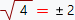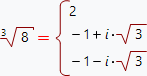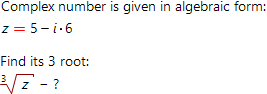# Complex number n-th root calculator, step by step solutionThis online calculator finds n-th root of the complex number with step by step solution. To find n-th root, first of all, one need to choose representation form (algebraic, trigonometric or exponential) of the initial complex number. Below we give some minimal theoretical background to be able to understand step by step solution given by our calculator.

According to the theory, n-th root of any number (nZ) has exactly n values. For example:More interenting example:where i - imaginary unit. As exercise, you can try to find third power of these values and make sure to get 8. There is a question: how to find all n values of n-th root of any given number? One should use the de Moivre's formula. So, complex number must be given in the trigonometric representation. Don't be worry, our online calculator automatically converts input data to trigonometric form as needed.

Complex number's root online calculator